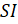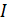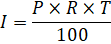### ARITHMETIC of FINANCE

Mathematics SSS 3 First Term

WEEK 3

ARITHMETIC of FINANCE

Performance Objectives

Student should be able to:

1. Calculate simple and compound interest.
2. Define and compute depreciation.
3. Define and compute annuity.

Content

Simple interest and Compound interest

Interest is paid on either money paid or borrowed. The principal is the initial amount of money deposited or borrowed.

When an interest is calculated and added to the principal at the end of an agreed time interval e.g. 3 months, 6 months, 1 year etc. Is called simple interest (or). This is given by the formulaWhere  View More